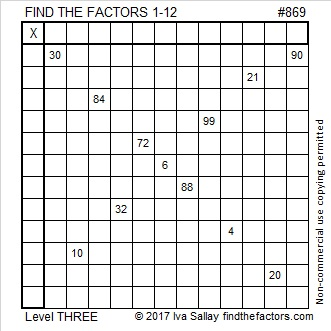# 869 and Level 3

8 – 6 + 9 = 11 so 869 is divisible by 11.

869 is equal to the sum of the 21 prime numbers from 5 to 83.Print the puzzles or type the solution on this excel file: 12 factors 864-874

• 869 is a composite number.
• Prime factorization: 869 = 11 × 79
• The exponents in the prime factorization are 1 and 1. Adding one to each and multiplying we get (1 + 1)(1 + 1) = 2 × 2 = 4. Therefore 869 has exactly 4 factors.
• Factors of 869: 1, 11, 79, 869
• Factor pairs: 869 = 1 × 869 or 11 × 79
• 869 has no square factors that allow its square root to be simplified. √869 ≈ 29.4788This site uses Akismet to reduce spam. Learn how your comment data is processed.